Documentation

# qammod

## Syntax

``y = qammod(x,M)``
``y = qammod(x,M,symOrder)``
``y = qammod(___,Name,Value)``

## Description

example

````y = qammod(x,M)` returns a baseband quadrature amplitude modulated (QAM) signal given input signal `x` and modulation order `M`.```

example

````y = qammod(x,M,symOrder)` returns a modulated signal and specifies the symbol order.```

example

````y = qammod(___,Name,Value)` specifies modulation behavior using `Name,Value` pairs and any of the previous syntaxes.```

## Input Arguments

collapse all

Input signal, specified as a scalar, vector, matrix, or 3-D array. The elements of x must be binary values or integers that range from 0 to (`M` – 1), where `M` is the modulation order.

### Note

To process input signal as binary elements, set the '`InputType`' name-value pair to `'bit'`. For binary inputs, the number of rows must be an integer multiple of log2(`M`). Groups of log2(`M`) bits are mapped onto a symbol, with the first bit representing the MSB and the last bit representing the LSB.

Data Types: `double` | `single` | `fi` | `int8` | `int16` | `uint8` | `uint16`

Modulation order, specified as a power-of-two scalar integer. The modulation order specifies the number of points in the signal constellation.

Example: `16`

Data Types: `double`

Symbol order, specified as `'gray'`, `'bin'`, or a vector.

• `'gray'` — Use Gray Code ordering

• `'bin'` — Use natural binary-coded ordering

• Vector — Use custom symbol ordering

Vectors must use unique elements whose values range from 0 to `M` – 1. The first element corresponds to the upper-left point of the constellation, with subsequent elements running down column-wise from left to right.

Example: [0 3 1 2]

Data Types: `char` | `double`

### Name-Value Pair Arguments

Specify optional comma-separated pairs of `Name,Value` arguments. `Name` is the argument name and `Value` is the corresponding value. `Name` must appear inside quotes. You can specify several name and value pair arguments in any order as `Name1,Value1,...,NameN,ValueN`.

Input type, specified as the comma-separated pair consisting of `'InputType'` and either `'integer'` or `'bit'`. If you specify `'integer'`, the input signal must consist of integers from 0 to `M` – 1. If you specify `'bit'`, the input signal must contain binary values, and the number of rows must be an integer multiple of log2(`M`).

Data Types: `char`

Unit average power flag, specified as the comma-separated pair consisting of `UnitAveragePower` and a logical scalar. When this flag is `true`, the function scales the constellation to an average power of 1 watt referenced to 1 ohm. When this flag is `false`, the function scales the constellation so that the QAM constellation points are separated by a minimum distance of 2.

Data Types: `logical`

Output data type, specified as the comma-separated pair consisting of `'OutputDataType'` and a numeric type object. See `numerictype` for more information on constructing these objects. If `OutputDataType` is omitted, the output data type is `double` for `double` or built-in integer inputs, and `single` for `single` inputs.

Option to plot constellation, specified as the comma-separated pair consisting of `'PlotConstellation'` and a logical scalar. To plot the QAM constellation, set `PlotConstellation` to `true`.

Data Types: `logical`

## Output Arguments

collapse all

Modulated signal, returned as a complex scalar, vector, matrix, or 3-D array. For integer inputs, output `y` has the same dimensions as input signal `x`. For bit inputs, the number of rows in `y` is the number of rows in `x` divided by log2(`M`).

Data Types: `double` | `single`

## Examples

collapse all

Modulate data using QAM and display the result in a scatter plot.

Set the modulation order to 16 and create a data vector containing each of the possible symbols.

```M = 16; x = (0:M-1)';```

Modulate the data using the `qammod` function.

`y = qammod(x,M);`

Display the modulated signal constellation using the `scatterplot` function.

`scatterplot(y)`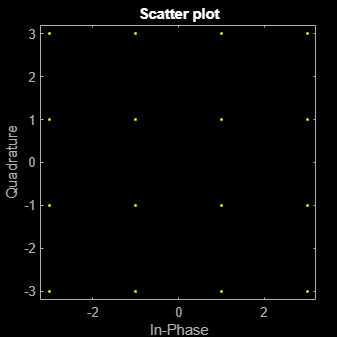Set the modulation order to 256, and display the scatter plot of the modulated signal.

```M = 256; x = (0:M-1)'; y = qammod(x,M); scatterplot(y)```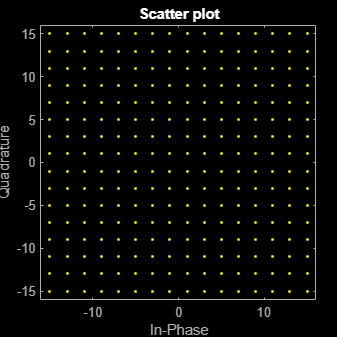Modulate random data symbols using QAM. Normalize the modulator output so that it has an average signal power of 1 W.

Set the modulation order and generate random data.

```M = 64; x = randi([0 M-1],1000,1);```

Modulate the data. Use the `'UnitAveragePower'` name-value pair to set the output signal to have an average power of 1 W.

`y = qammod(x,M,'UnitAveragePower',true);`

Confirm that the signal has unit average power.

`avgPower = mean(abs(y).^2)`
```avgPower = 1.0070 ```

Plot the resulting constellation.

```scatterplot(y) title('64-QAM, Average Power = 1 W')```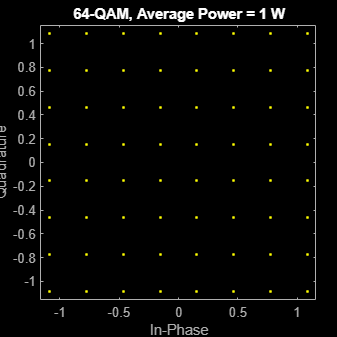Plot QAM constellations for Gray, binary, and custom symbol mappings.

Set the modulation order, and create a random data sequence.

```M = 16; d = randi([0 M-1],1000,1);```

Modulate the data, and plot its constellation.

`y = qammod(d,M,'PlotConstellation',true);`The default symbol mapping uses Gray ordering. The ordering of the points is not sequential.

Repeat the modulation process with binary symbol mapping.

`z = qammod(d,M,'bin','PlotConstellation',true);`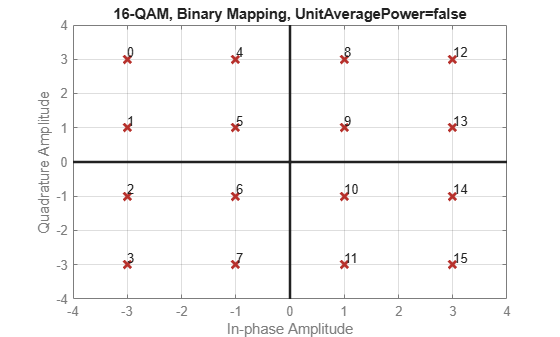The symbol mapping follows a natural binary order and is sequential.

Create a custom symbol mapping.

`smap = randperm(16)-1;`

Modulate and plot the constellation.

`w = qammod(d,M,smap,'PlotConstellation',true);`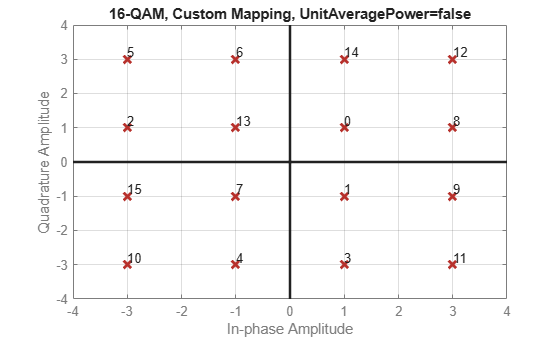Modulate a sequence of bits using 64-QAM. Pass the signal through a noisy channel. Display the resultant constellation diagram.

Set the modulation order, and determine the number of bits per symbol.

```M = 64; k = log2(M); ```

Create a binary data sequence. When using binary inputs, the number of rows in the input must be an integer multiple of the number of bits per symbol.

```data = randi([0 1],1000*k,1); ```

Modulate the signal using bit inputs, and set it to have unit average power.

```txSig = qammod(data,M,'InputType','bit','UnitAveragePower',true); ```

Pass the signal through a noisy channel.

```rxSig = awgn(txSig,25); ```

Plot the constellation diagram.

```cd = comm.ConstellationDiagram('ShowReferenceConstellation',false); step(cd,rxSig) ```Demodulate a fixed-point QAM signal and verify that the data is recovered correctly.

Set the modulation order, and determine the number of bits per symbol.

```M = 64; bitsPerSym = log2(M);```

Generate random bits. When operating in bit mode, the length of the input data must be an integer multiple of the number of bits per symbol.

`x = randi([0 1],10*bitsPerSym,1);`

Modulate the input data using a binary symbol mapping. Set the modulator to output fixed-point data. The numeric data type is signed with a 16-bit word length and a 10-bit fraction length.

```y = qammod(x,M,'bin','InputType','bit','OutputDataType', ... numerictype(1,16,10));```

Demodulate the 64-QAM signal. Verify that the demodulated data matches the input data.

```z = qamdemod(y,M,'bin','OutputType','bit'); s = isequal(x,double(z))```
```s = logical 1 ```

collapse all

### Gray Code

A Gray code, also known as a reflected binary code, is a system where the bit patterns in adjacent constellation points differ by only one bit.

## Compatibility Considerations

expand all

Errors starting in R2018b